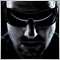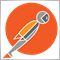# ++/-- Illegal operation use on operator overloading ... Why?1220

Can someone help here ... the operator is overloaded, actually it should work

```//+------------------------------------------------------------------+
//|                                                     PlusPlus.mq5 |
//+------------------------------------------------------------------+

struct STest
{
double value;

double ToDouble()               { return value; }
//--- Assignment operators
void operator =  (STest &dec)   { value=dec.ToDouble(); }
void operator =  (double v)     { value=v; }

//--- Logical operators
bool operator == (STest &dec)   { return (value==dec.value); }
bool operator == (double v)     { return (value==v); }
bool operator != (STest &dec)   { return (value!=dec.value); }
bool operator != (double v)     { return (value!=v); }
bool operator >= (STest &dec)   { return (value>=dec.value); }
bool operator >= (double v)     { return (value>=v); }
bool operator <= (STest &dec)   { return (value<=dec.value); }
bool operator <= (double v)     { return (value<=v); }
bool operator >  (STest &dec)   { return (value>dec.value); }
bool operator >  (double v)     { return (value>v); }
bool operator <  (STest &dec)   { return (value<dec.value); }
bool operator <  (double v)     { return (value<v); }

//--- Arithmetic & binary operators
void operator -= (STest &dec)   { value-=dec.value; }
void operator -= (double v)     { value-=v; }
void operator += (STest &dec)   { value+=dec.value; }
void operator += (double v)     { value+=v; }
void operator *= (STest &dec)   { value*=dec.value; }
void operator *= (double v)     { value*=v; }
void operator /= (STest &dec)   { value/=dec.value; }
void operator /= (double v)     { value/=v; }
void operator ++ (void)         { value+=1; }
void operator -- (void)         { value-=1; }
};

//+------------------------------------------------------------------+
//| Expert initialization function                                   |
//+------------------------------------------------------------------+
int OnInit()
{

STest t;
t=1;
t++;        // Illegal operation use ... why?

//---
return(INIT_FAILED);
}
```38626

Doerk Hilger:

Can someone help here ... the operator is overloaded, actually it should work

Because you didn't define the overload correctly. You need to return an unchanged STest value.
```         STest operator++(int)          { STest tmp; tmp=this; value+=1; return(tmp); }
```19422

1. `         void operator ++ (void)         { value+=1; }`
That is operator ++t.

2. Alain gave you t++. Alternatively implement postfix using prefix:
`         STest operator++(int)          { STest tmp=this; ++this; return tmp; }`
That is why you should prefer ++x over x++38626

William Roeder:
1. That is operator ++t.

Yes but incorrectly defined as it can't be assigned. I don't think it's possible to define it correctly with a struct as a pointer should be returned.19422

Alain Verleyen: Yes but incorrectly defined as it can't be assigned. I don't think it's possible to define it correctly with a struct as a pointer should be returned.

Agreed. If it was a class, I'd have a dozen additional comments as const correctness, and returning pointer to self for chaining, etc. But it's not, so I didn't.1220

Thx guys.1220

By the way, is there a possibility to get this done with overloading / cast operator overloading?

```STest t;
double v=t;     // "=" Illegal operation```38626

Doerk Hilger:

By the way, is there a possibility to get this done with overloading / cast operator overloading?

I don't think it's possible currently in mql.19422

That would require operator double(). Not listed at: MQL5 Reference → Language Basics → Functions → Operation Overloading2383

Alain Verleyen:
Because you didn't define the overload correctly. You need to return an unchanged STest value.

This is only partially correct. You should not return a value from a self mutating operator. As such, the operator should be define as follows:

```struct STest {
double value;
STest():value(0.0){}
STest(double n) {
this.value = n;
}
void operator ++ (int) {
this.value += 1.0;
}
};
//+------------------------------------------------------------------+
void OnStart() {
STest t = 1;
t++;
Print(t.value);
}```
To add comments, please log in or register